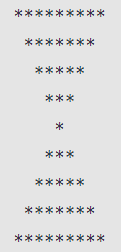Related Tags

javascript
communitycreator

# How to create an hourglass star pattern in JavaScript

Ayush Trivedi

### Overview

In this shot, we will learn how to create an hourglass star pattern using JavaScript. This pattern is more complex than other patterns. The logic to create an hourglass pattern is creating a downside pyramid on an upside pyramid.

An hourglass star pattern looks like the image below:### Code

let n = 5;
// downside pyramid
for (let i = 0; i < n ; i++) {
// printing spaces
for (let j = 0; j < i; j++) {
process.stdout.write(' ');
}
// printing star
for (let k = 0; k < (n - i) * 2 - 1; k++) {
process.stdout.write('*');
}
console.log();
}

// Upside pyramid
for (let i = 2; i <= n; i++) {
// printing spaces
for (let j = 1; j <= n - i; j++) {
process.stdout.write(' ')
}
// printing star
for (let k = 0; k < 2 * i - 1; k++) {
process.stdout.write('*')
}
console.log();
}

### Code explanation

• In line 1, we initialize a number that is the height (rows) of the downside pyramid (i.e., 5) and one less for the upside pyramid (i.e., 4), so the total row number (height) for the hourglass is 9.

#### Downside pyramid (lines 3-13)

• In line 3, we have our first outer for loop, which will iterate over the height of the downside triangle (that is 5, n=5).

• In line 5, we have the first inner for loop to print spaces for i times, where i is the outer loop iteration number. If i=0, i.e., for the st row, the number of spaces before the star is 0, for i=3 (4th row), the space is 3, and so on.

• In line 6, the standard method of JavaScript is used to print the space (’ '). process.stdout.write() will print the space.

• In line 9, we have the second nested for loop to print * for (n - i) * 2 - 1 times, where i is the current iteration number of the outer loop. If i=3, i.e., for the 4th row after 3 spaces it will print 3 stars (5 - 3) * 2 - 1.

• In line 10, the standard method of JavaScript is used to print the space (*). process.stdout.write() will print the space.

• In line 12, we used console.log() with null, as it will change to a new line. We can use process.stdout.write(’\n’) to change the line.

#### Upside pyramid (lines 16-26)

• In line 16, we have our outer for loop, which will iterate from i=2 to i=n=5. We are initializing i from 2, as we need to skip the first row, which will print only one star in the middle. This is already done by the above pattern (downward pyramid)

• In line 18, we have the first nested for loop to print spaces for i+1 times, where i is the outer loop iteration number. If i=3, i.e., for the second row, the number of spaces before the star is 3, and so on.

• In line 22, we have the second inner for loop to print * for 2 * i - 1 times, where i is the current iteration number of the outer loop. If i=3, i.e., for the second row, after 3 spaces it will print 5(2 * 3 - 1) star.

• In line 23, the standard method of JavaScript is used to print the space (*). process.stdout.write() will print the space.

• In line 25, we used console.log() with null, as it will change to a new line. We can use process.stdout.write(’\n’) to change the line.

### Output

We have completed the task of creating an hourglass star pattern in JavaScript.

RELATED TAGS

javascript
communitycreator

CONTRIBUTOR

Ayush Trivedi
RELATED COURSES

View all Courses

Keep Exploring

Learn in-demand tech skills in half the time